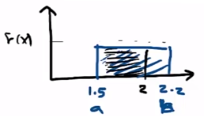Need Help?

Subscribe to Probability

###### \${selected_topic_name}
• Notes
• Comments & Questions

The net weight in pounds of a packaged chemical her-
bicide is uniform for $49.75<x<50.25$ pounds.

(a) Determine the mean and variance of the weight of pack-
ages.
(b) Determine the cumulative distribution function of the
weight of packages.
(c) Determine $P(X<50.1)$ .

$49.75<x<50.25$

(a) $\mu, \sigma^{2}$

(b) $F(x)$

(c) $P(x<50.1)$

(a) $\mu=\frac{b+a}{2}, \sigma^{2}=\frac{(b-a)^{2}}{12}$

$\mu=\frac{50.25+49.75}{2}=50$

$\sigma^{2}=\frac{(50.25-49.75)^{2}}{12}=0.0208$

(b) $F(x)=\int_{a}^{x} \frac{1}{b-a} d x=\frac{x-a}{b-a}$

$a \leq x < b$

$49.75<x<50.25$

$F(x)=\frac{x-49.75}{50.25-49.75}=2 x-99.5$

$F(x)=\left\{\begin{array}{cc}{0} & {x<49.75} \\ {2 x-99.5} & {49.75<x<50.25} \\ {1} & {50.25<x}\end{array}\right.$

$P(x<50.1)=F(50.1)$

$=2(50.1)-99.5=0.7$

Suppose the time it takes a data collection operator to
fill out an electronic form for a database is uniformly between
1.5 and 2.2 minutes.

(a) What is the mean and variance of the time it takes an op-
erator to fill out the form?
(b) What is the probability that it will take less than two min-
utes to fill out the form?
(c) Determine the cumulative distribution function of the time
it takes to fill out the form.

$1.5<x<2.2$

(a) $\mu, \sigma^{2}$

(b) $P(x<2)$

(c) $F(x)$$\mu=\frac{b+a}{2}=\frac{2.2+1.5}{2}=1.85$

$\sigma^{2}=\frac{(2.2-1.5)^{2}}{12}=0.0408$

(b) $P(x<2)$

$F(x)=\frac{1}{b-a}$

$\int_{a}^{2} F(x) d x=\int_{1.5}^{2} \frac{1 d x}{2.2-1.5}$

$=\int_{1.5}^{2} 1.4286 d x$

$P(x<2)=\left.1.4286 x\right|_{1.5} ^{2}=0.7143$

(c) $F(x) \rightarrow a<x<b$

$F(x)=\frac{x-a}{b-a}=\frac{x-1.5}{0.7}=1.4286 x-2.143$

$F(x)=\left\{\begin{array}{cc}{0} & {x<1.5} \\ {1.4286 x-2.143} & {1.5<x<2.2} \\ {1} & {2.2 < x}\end{array}\right.$

$p(x<2)=F(2)$

substitute in

$1.4286 x-2.143 \quad 1.5<x<2.2$

$=0.7143$

No comments yet